# Interpolation on Graphene deflector scan¶

Simple workflow for the interpolation of data along a generic path in the k-space from its isoenergy cuts. The data are a deflector scan on graphene as simulated from a third nearest neighbor tight binding model. The same workflow can be applied to any tilt-, polar-, deflector- or hv-scan.

Import the “fundamental” python libraries for a generic data analysis:

import numpy as np
import matplotlib.pyplot as plt


# from navarp.utils import navfile
# file_name = r"nxarpes_simulated_cone.nxs"


Here we build the simulated graphene signal with a dedicated function defined just for this purpose:

from navarp.extras.simulation import get_tbgraphene_deflector

entry = get_tbgraphene_deflector(
scans=np.linspace(-5., 20., 91),
angles=np.linspace(-25, 6, 400),
ebins=np.linspace(-13, 0.4, 700),
tht_an=-18,
phi_an=0,
hv=120,
gamma=0.05
)


## Fermi level autoset¶

entry.autoset_efermi(scan_range=[-5, 5], energy_range=[115.2, 115.8])
print("Energy of the Fermi level = {:.0f} eV".format(entry.efermi))
print("Energy resolution = {:.0f} meV".format(entry.efermi_fwhm*1000))

entry.plt_efermi_fit()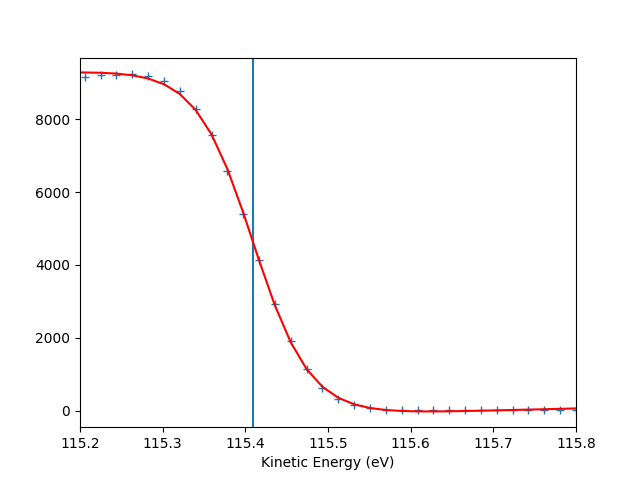Out:

Fermi level at 115.4095 eV
Energy resolution = 137.5 meV (i.e. FWHM of the Gaussian shape which, convoluted with a step function, fits the Fermi edge)
Photon energy is now set to 120.0095 eV (instead of 120.0000 eV)
Energy of the Fermi level = 115 eV
Energy resolution = 137 meV


## Check for the Fermi level alignment¶

entry.isoscan(scan=0, dscan=0).show(yname='eef')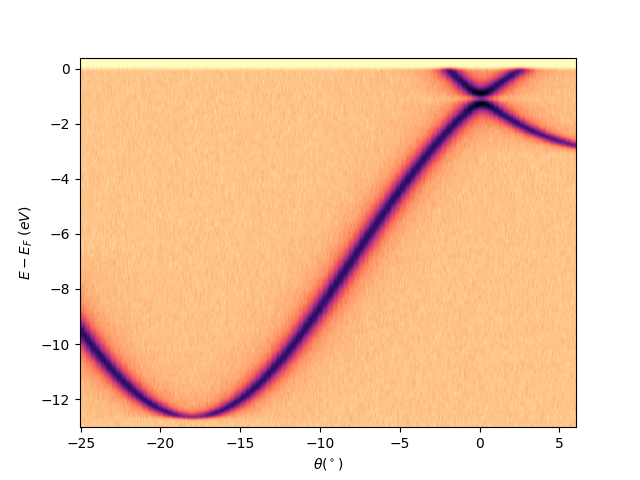Out:

<matplotlib.collections.QuadMesh object at 0x7f88bfdcb210>


## Plotting iso-energetic cut at ekin = efermi¶

entry.isoenergy(0, 0.02).show()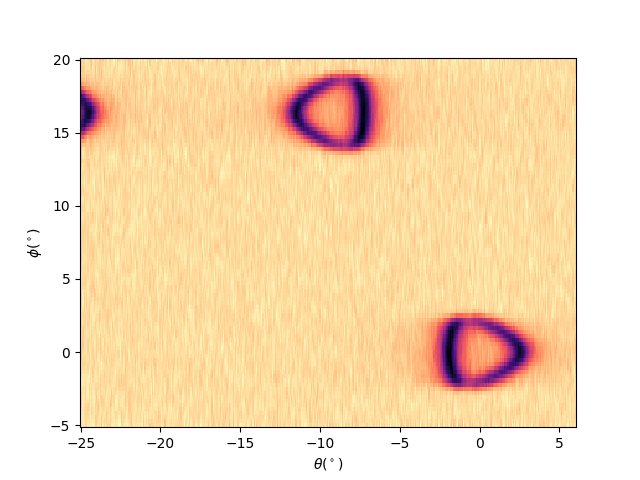Out:

<matplotlib.collections.QuadMesh object at 0x7f88bff3c250>


## Set the k-space for the transformation¶

entry.set_kspace(
tht_p=0.1,
k_along_slit_p=1.7,
scan_p=0,
ks_p=0,
e_kin_p=114.3,
)


Out:

tht_an = -17.979
scan_type =  deflector
inn_pot = 14.000
scans_0 = 0.000
phi_an = 0.000


and check the isoenergy at the Fermi level:

entry.isoenergy(0, 0.02).show()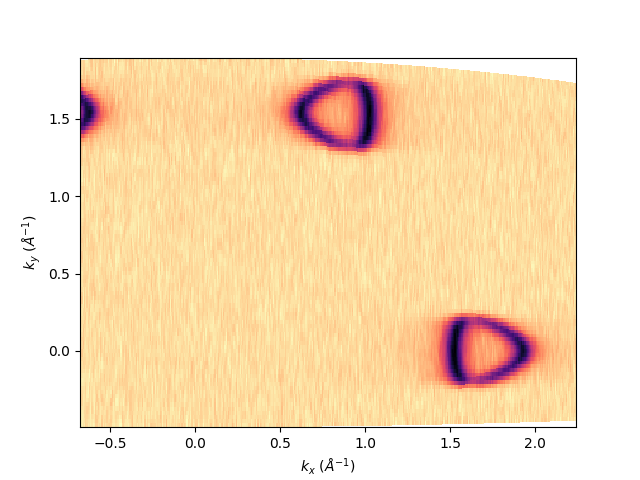Out:

<matplotlib.collections.QuadMesh object at 0x7f88bf196f90>


## Define the interpolation path points¶

kbins = 900
k_GK = 1.702
k_pts_xy = np.array([
[0, 0],
[k_GK, 0],
[k_GK*np.cos(np.pi/3), k_GK*np.sin(np.pi/3)+0.05],
[0, 0]
])
kx_pts = k_pts_xy[:, 0]
ky_pts = k_pts_xy[:, 1]

klabels = [
r'$\Gamma$',
r'$\mathrm{K}$',
r'$\mathrm{K}^{\prime}$',
r'$\Gamma$'
]


and show them on the isoenergy at the Dirac point energy:

entry.isoenergy(-1.1, 0.02).show()
plt.plot(kx_pts, ky_pts, '-+')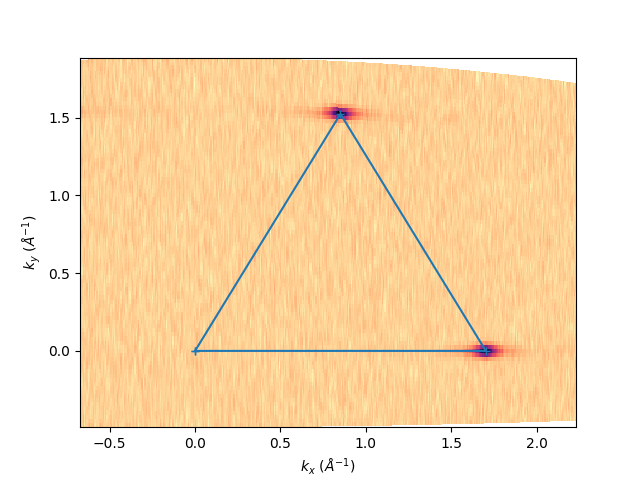Out:

[<matplotlib.lines.Line2D object at 0x7f88bfeb8510>]


Run the interpolation defining an isok:

isok = entry.isok(kx_pts, ky_pts, klabels)


Show the final results with the executed path on the isoenergy: sphinx_gallery_thumbnail_number = 6

fig, axs = plt.subplots(1, 2)

entry.isoenergy(0, 0.02).show(ax=axs)

isok.path_show(axs, 'k', 'k', xytext=(8, 8))

qmesh = isok.show(ax=axs)

fig.tight_layout()
fig.colorbar(qmesh)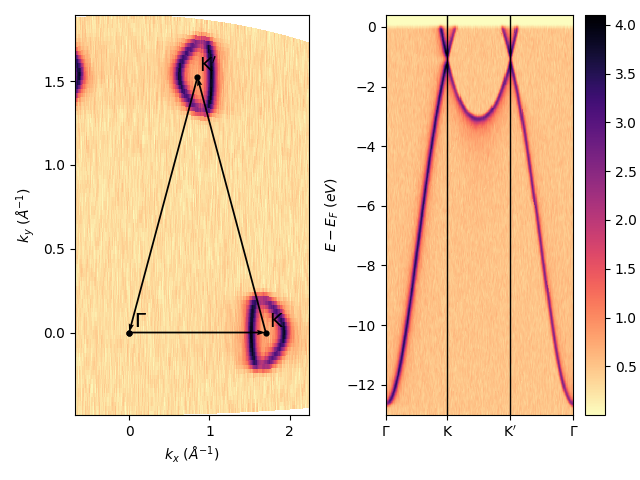Out:

<matplotlib.colorbar.Colorbar object at 0x7f88bfc75110>


Total running time of the script: ( 0 minutes 16.476 seconds)

Gallery generated by Sphinx-Gallery